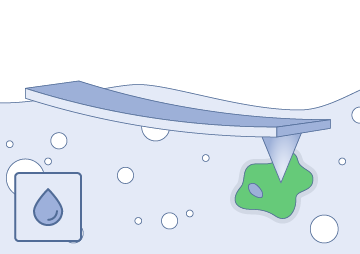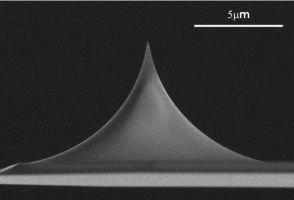## Fluid Tapping / AC AFM Probes### Most popular in this categoryqp-BioAC
• uniform quality SPM probe
• XY-auto alignment with Alignment Chip
• 3 cantilevers
• C1 = 0.3 N/m; fo1 = 90 kHz
• C2 = 0.1 N/m; fo2 = 50 kHz
• C3 = 0.06 N/m; fo3 = 30 kHz

### uniqprobe™qp-BioT
• uniform quality SPM probe
• XY-auto alignment with Alignment Chip
• 2 cantilevers
• C1 = 0.3 N/m; fo1 = 50 kHz
• C2 = 0.08 N/m; fo2 = 20 kHz
qp-BioAC
• uniform quality SPM probe
• XY-auto alignment with Alignment Chip
• 3 cantilevers
• C1 = 0.3 N/m; fo1 = 90 kHz
• C2 = 0.1 N/m; fo2 = 50 kHz
• C3 = 0.06 N/m; fo3 = 30 kHz
qp-BioAC-CI
• uniform quality SPM probe
• special rounded tip for live cell imaging
• XY-auto alignment with Alignment Chip
• 3 cantilevers
• C1 = 0.3 N/m; fo1 = 90 kHz
• C2 = 0.1 N/m; fo2 = 50 kHz
• C3 = 0.06 N/m; fo3 = 30 kHz
qp-SCONT
• uniform quality SPM probe
• XY-auto alignment with Alignment Chip
• Contact Mode
• C = 0.01 N/m; fo = 11 kHz
qp-CONT
• uniform quality SPM probe
• XY-auto alignment with Alignment Chip
• Contact Mode
• C = 0.1 N/m; fo = 30 kHz